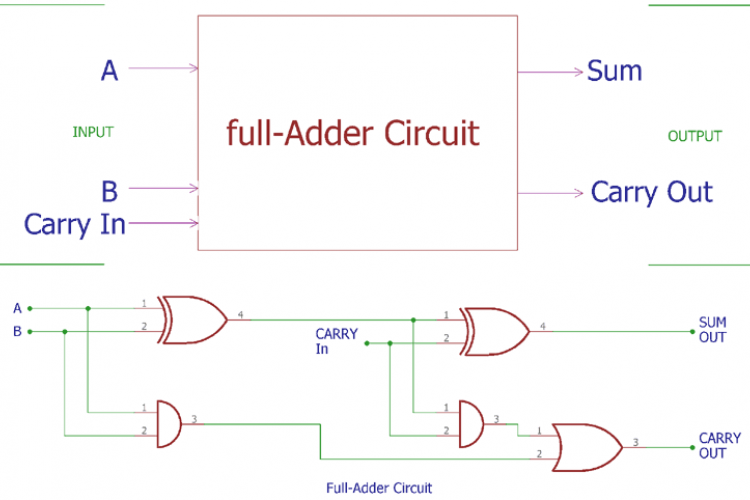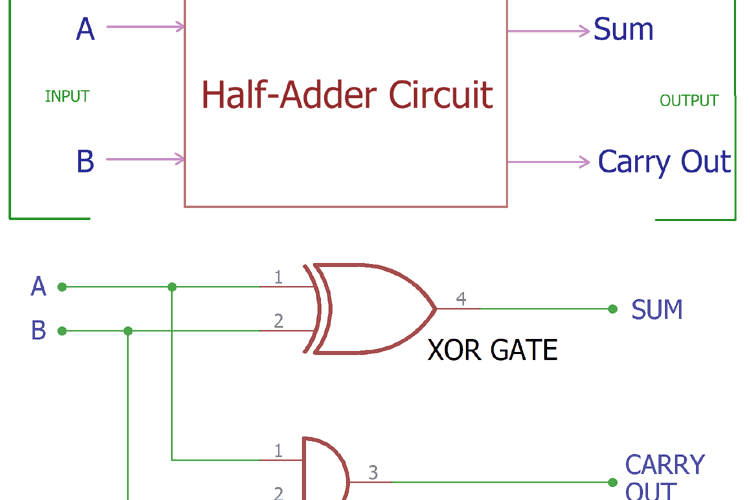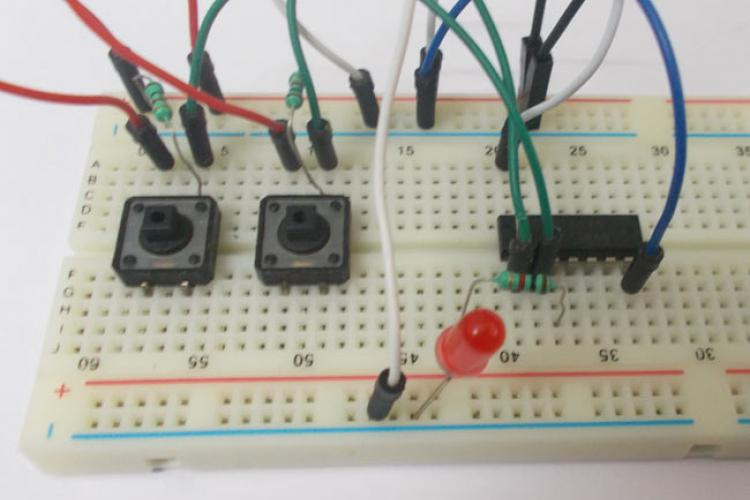# EX ORFull Adder Circuit and its ConstructionIn previous tutorial of half adder circuit construction, we had seen how computer uses single bit binary numbers 0 and 1 for…Half Adder Circuit and its ConstructionComputer uses binary numbers 0 and 1. An adder circuit uses these binary numbers and calculates the addition. A binary adder…XOR GateXOR or EX-OR gate is a digital logic gate, designed for arithmetic and logical operations, Every electronic student must have…